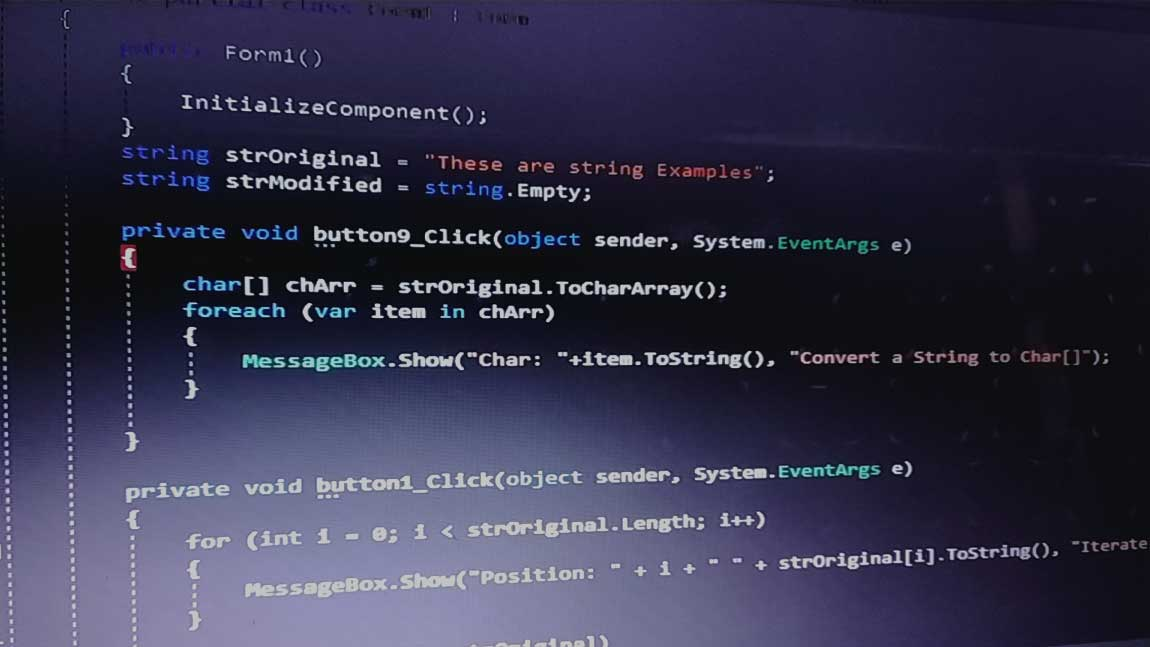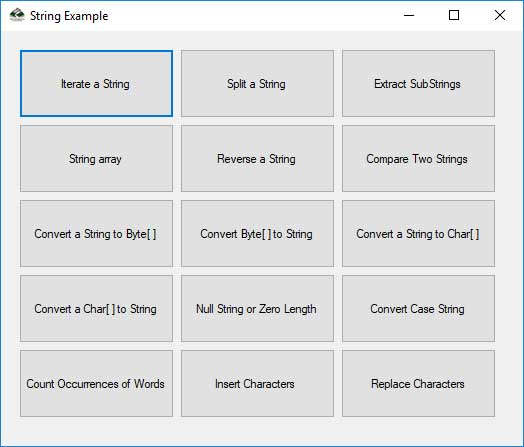# CSharp Common String Operations Part-I

• Home -
• Blog -
• CSharp Common String Operations Part-I• 28 May
• 2019

## CSharp Common String Operations Part-I

We have compiled some common string operations in this article that we encounter while working with the string class. We covered 15 common string operations in Part I. We're going to continue this article in the next article and cover 15 more.

First we declared two string variables strOriginal and strModified. All the examples are based on these two pre-declared string variables.

C#

``````
string strOriginal = "These are string Examples";
string strModified = string.Empty;

``````

VB.Net

``````
Dim strOriginal As String = "These are string Examples"
Dim strModified As String = string.Empty

``````

We can use the "for loop" or "foreach loop" to iterate through a string. The "for loop" gives us more flexibility over the iteration.

For Example:

For Loop in C#

``````
for (int i = 0; i < strOriginal.Length; i++)
{
MessageBox.Show(strOriginal[i].ToString());
}

``````

For Each Loop in C#

``````
foreach (char c in strOriginal)
{
MessageBox.Show(c.ToString());
}

``````

For Loop in VB.Net

``````
For i As Integer = 0 To strOriginal.Length - 1
MessageBox.Show(strOriginal(i).ToString())
Next i

``````

For Each Loop in VB.Net

``````
For Each c As Char In strOriginal
MessageBox.Show(c.ToString())
Next c

``````

`String.Split()` can be used to split strings. The method takes a array of chars that represent characters that are to be used as delimiters. We're going to split the strOriginal string using 'space' as a delineator.

Split string in C#

``````
char[] del = {' '};
string[] strArr = strOriginal.Split(del);
foreach (string s in strArr)
{
MessageBox.Show(s);
}

``````

Split string in VB.Net

``````
Dim delim As Char() = {" "c}
Dim strArr As String() = strOriginal.Split(delim)
For Each s As String In strArr
MessageBox.Show(s)
Next s

``````

The `String.Substring()` gets a substring from a string starting from a specified position of the character. We can specify the length, too.

SubStrings in C#

``````
// only starting position specified
strModified = strOriginal.Substring(10);
MessageBox.Show(strModified);

// starting position and length of string to be extracted specified
strModified = strOriginal.Substring(10, 3);
MessageBox.Show(strModified);

``````

SubStrings in VB.Net

``````
' only starting position specified
strModified = strOriginal.Substring(10)
MessageBox.Show(strModified)

' starting position and length of string to be extracted specified
strModified = strOriginal.Substring(10, 3)
MessageBox.Show(strModified)

``````

We can create different type of string array. There are  two type of string array Single Dimensional and Multi Dimensional arrays. Let's look at some of them

Single Dimensional String Array in C#

``````
// Single Dimensional String Array

string[] strArr = new string { "string 1", "string 2", "string 3"};
// Omit Size of Array
string[] strArr1 = new string[] { "string 1", "string 2", "string 3" };
// Omit new keyword
string[] strArr2 = {"string 1", "string 2", "string 3"};

``````

Multi Dimensional String Array in C#

``````
// Multi Dimensional String Array

string[,] strArr3 = new string[2, 2] { { "string 1", "string 2" }, { "string 3", "string 4" } };
// Omit Size of Array
string[,] strArr4 = new string[,] { { "string 1", "string 2" }, { "string 3", "string 4" } };
// Omit new keyword
string[,] strArr5 = { { "string 1", "string 2" }, { "string 3", "string 4" } };

``````

Single Dimensional String Array in VB.Net

``````
' Single Dimensional String Array

Dim strArr As String() = New String(2) { "string 1", "string 2", "string 3"}
' Omit Size of Array
Dim strArr1 As String() = New String() { "string 1", "string 2", "string 3" }
' Omit new keyword
Dim strArr2 As String() = {"string 1", "string 2", "string 3"}

``````

Multi Dimensional String Array in VB.Net

``````
' Multi Dimensional String Array

Dim strArr3 As String(,) = New String(1, 1) { { "string 1", "string 2" }, { "string 3", "string 4" } }
' Omit Size of Array
Dim strArr4 As String(,) = New String(, ) { { "string 1", "string 2" }, { "string 3", "string 4" } }
' Omit new keyword
Dim strArr5 As String(,) = { { "string 1", "string 2" }, { "string 3", "string 4" } }

``````

Using the `StrReverse()` function is one of the simplest ways to reverse a string. We must add a reference to the Microsoft.VisualBasic dll to use it in C#.

Reverse a String in C#

``````
string strModified = Microsoft.VisualBasic.Strings.StrReverse(strOriginal);
MessageBox.Show(strModified);

``````

Reverse a String in VB.Net

``````
Dim strModified As String = StrReverse(strOriginal)
MsgBox(strModified)

``````

We can compare two strings using the String.Compare() methos. The third parameter is Boolean, which determines whether or not the search is case sensitive.

Compare Two String in C#

``````
if ((string.Compare(strOriginal, strModified, false)) < 0)
{
MessageBox.Show("strOriginal is less than strOriginal1");
}
else if ((string.Compare(strOriginal, strModified, false)) > 0)
{
MessageBox.Show("strOriginal is more than strOriginal1");
}
else if ((string.Compare(strOriginal, strModified, false)) == 0)
{
MessageBox.Show("Both strings are equal");
}

``````

Compare Two String in VB.Net

``````
If (String.Compare(strOriginal, strModified, False)) < 0 Then
MessageBox.Show("strOriginal is less than strOriginal1")
ElseIf (String.Compare(strOriginal, strModified, False)) > 0 Then
MessageBox.Show("strOriginal is more than strOriginal1")
ElseIf (String.Compare(strOriginal, strModified, False)) = 0 Then
MessageBox.Show("Both strings are equal")
End If

``````

The `Encoding.GetBytes()` encodes all the characters into a sequence of bytes. The method consists of six overloads from which we use the. GetBytes(string) encoding.

Convert string in Byte[] in C#

``````
byte[] b = Encoding.Unicode.GetBytes(strOriginal);

``````

Convert string in Byte[] in VB.Net

``````
Dim b As Byte() = Encoding.Unicode.GetBytes(strOriginal)

``````

Note: Depending upon your requirement, we can adopt various encoding schemes (ASCII, Unicode, etc.).

The `Encoding.GetString()` decodes a sequence of bytes into a string.

Convert Byte[] to string in c#

``````
// Assuming you have a Byte Array byte[] b
strModified = Encoding.Unicode.GetString(b);

``````

Convert Byte[] to string in VB.Net

``````
' Assuming you have a Byte Array byte[] b
strModified = Encoding.Unicode.GetString(b)

``````

Use the `String.ToCharArray()` which copies the characters in the string to Unicode character array, to convert a string to char array.

Convert a string to char[] in C#

``````
char[] chArr = strOriginal.ToCharArray();

``````

Convert a string to char[] in VB.Net

``````
Dim chArr As Char() = strOriginal.ToCharArray()

``````

The String Constructor which accepts a character array is an easy way to convert a character array to a string

Convert a Char[] to string C#

``````
strModified = new String(chArr);

``````

Convert a Char[] to string VB.Net

``````
strModified = New String(chArr)

``````

The `String.IsNullOrEmpty(String)`, which returns a boolean value, shall be used if a string is null or empty.

String is null or Zero Length in C#

``````
bool check = string.IsNullOrEmpty(strOriginal);

``````

String is null or Zero Length in C#

``````
Dim check As Boolean = String.IsNullOrEmpty(strOriginal)

``````

The Class String contains methods for converting a string into lower and upper cases. However, a method for converting a string to a suitable case / title case is missing. We are therefore going to do the same with the `TextInfo` class.

Convert the case of string in C#

``````
System.Globalization.TextInfo textInfo = cultureInfo.TextInfo;
// Lower Case
MessageBox.Show(textInfo.ToLower(strOriginal));
// Upper Case
MessageBox.Show(textInfo.ToUpper(strOriginal));
// Proper Case
MessageBox.Show(textInfo.ToTitleCase(strOriginal));

``````

Convert the case of string in VB.Net

``````
Dim textInfo As System.Globalization.TextInfo = cultureInfo.TextInfo
' Lower Case
MessageBox.Show(textInfo.ToLower(strOriginal))
' Upper Case
MessageBox.Show(textInfo.ToUpper(strOriginal))
' Proper Case
MessageBox.Show(textInfo.ToTitleCase(strOriginal))

``````

We can take several ways to find a word's occurrence in a string.One of the ways to find the occurence of the word is by using the `String.IndexOf()`. `String.InStr()` is used in VB.NET.

Another easy way to use Regex.Matches() collection's `Counter` property. But this is a slow procedure. These two methods are to be explored in the sample.

Count the occurrence of word in C#

``````
// Using IndexOf
int strt = 0;
int cnt = -1;
int idx = -1;
strOriginal = "Uttarakhand, a state in northern India";
string srchString = "state";
while (strt != -1)
{
strt = strOriginal.IndexOf(srchString, idx + 1);
cnt += 1;
idx = strt;
}
MessageBox.Show(srchString + " occurs " + cnt + " times");

// Using Regular Expression
System.Text.RegularExpressions.Regex rex = new System.Text.RegularExpressions.Regex(srchString);
int count = rex.Matches(strOriginal).Count;
MessageBox.Show(srchString + " occurs " + count + " times");

``````

Count the occurrence of word in VB.Net

``````
' Using IndexOf
Dim strt As Integer = 0
Dim cnt As Integer = -1
Dim idx As Integer = -1
strOriginal = "Uttarakhand, a state in northern India"
Dim srchString As String = "state"
Do While strt <> -1
strt = strOriginal.IndexOf(srchString, idx + 1)
cnt += 1
idx = strt
Loop
MessageBox.Show(srchString & " occurs " & cnt & " times")

' Using Regular Expression
Dim rex As System.Text.RegularExpressions.Regex = New System.Text.RegularExpressions.Regex(srchString)
Dim count As Integer = rex.Matches(strOriginal).Count
MessageBox.Show(srchString & " occurs " & count & " times")

``````

In a certain Index location, the `String.insert()` inserts text. At a given index position we can insert any character or string. For example: we insert "very" in the string strOriginal at index 26.

Insert characters inside string in C#

``````
strModified = strOriginal.Insert(10, "common");
MessageBox.Show(strModified);

``````

Insert characters inside string in VB.Net

``````
strModified = strOriginal.Insert(10, "common")
MessageBox.Show(strModified)

``````

String.Replace() removes strings and substitutes them with a new character or string.

Replace character in string in C#

``````
strModified = strOriginal.Replace("are", "are common");
MessageBox.Show(strModified);

``````

Replace character in string in VB.Net

``````
strModified = strOriginal.Replace("are", "are common")
MessageBox.Show(strModified)

``````

We will explore several other string operations commonly used in projects in the following article.